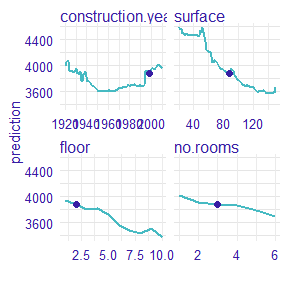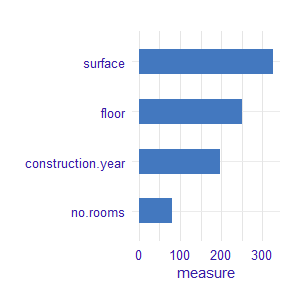# Example with random forest model

## Example with random forest regression model

In this vignette we present measure for random forest regression model.

### 1 Dataset

We work on Apartments dataset from DALEX package.

#>   m2.price construction.year surface floor no.rooms    district
#> 1     5897              1953      25     3        1 Srodmiescie
#> 2     1818              1992     143     9        5     Bielany
#> 3     3643              1937      56     1        2       Praga
#> 4     3517              1995      93     7        3      Ochota
#> 5     3013              1992     144     6        5     Mokotow
#> 6     5795              1926      61     6        2 Srodmiescie

### 2 Random forest regression model

Now, we define a random forest regression model and use explain from DALEX.

library("randomForest")
apartments_rf_model <- randomForest(m2.price ~ construction.year + surface + floor +
no.rooms, data = apartments)
explainer_rf <- explain(apartments_rf_model,
data = apartmentsTest[,2:5], y = apartmentsTest\$m2.price)

### 3 New observation

We need to specify an observation. Let consider a new apartment with following attributes. Moreover, we calculate predict value for this new observation.

new_apartment <- data.frame(construction.year = 1998, surface = 88, floor = 2L, no.rooms = 3)
predict(apartments_rf_model, new_apartment)
#>        1
#> 3885.451

### 4 Calculate Ceteris Paribus profiles

Let see the Ceteris Paribus Plots calculated with ceteris_paribus() function.

library("ingredients")
profiles <- ingredients::ceteris_paribus(explainer_rf, new_apartment)
plot(profiles) + show_observations(profiles)### 5 Calculate measure of local variable importance

Now, we calculated a measure of local variable importance via oscillation based on Ceteris Paribus plot. We use variant with all parameters equals to TRUE.

library("vivo")
measure <- local_variable_importance(profiles, apartments[,2:5],
absolute_deviation = TRUE, point = TRUE, density = TRUE)
plot(measure)For the new observation the most important variable is surface, then floor, construction.year and no.rooms.